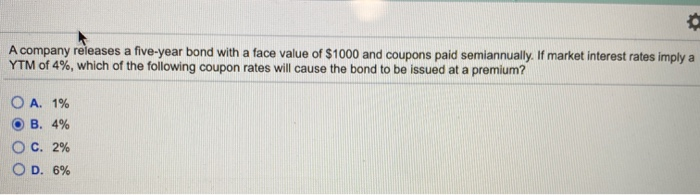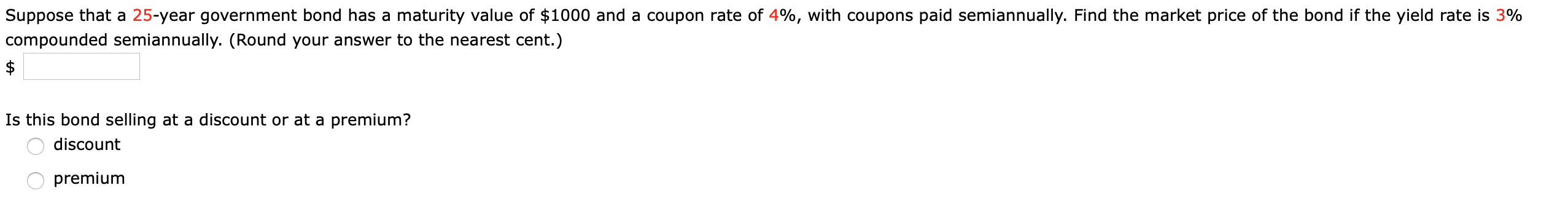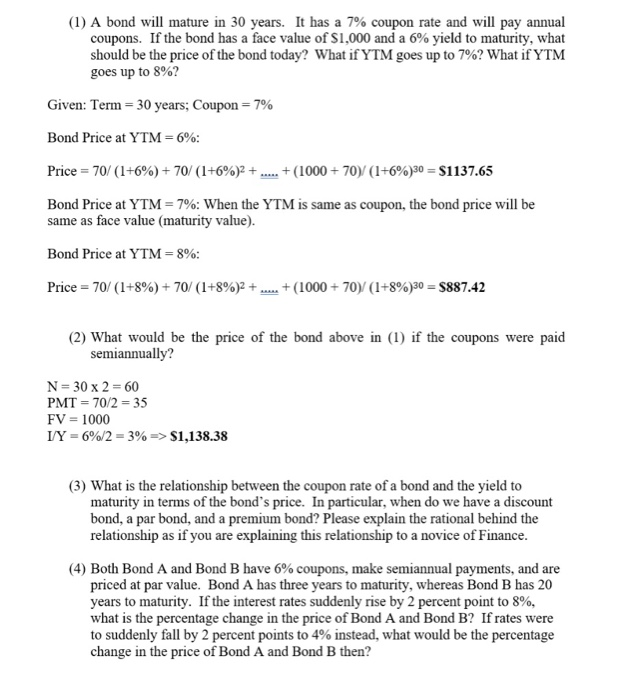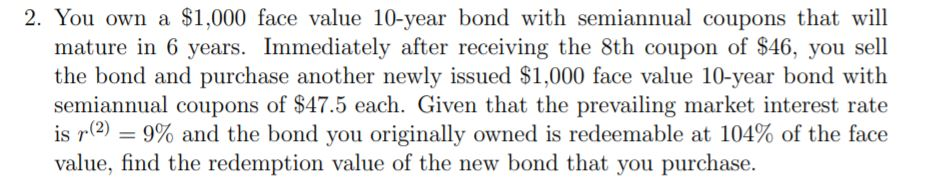Question

# A company releases a five-year bond with a face value of \$1000 and coupons paid semiannually....A company releases a five-year bond with a face value of \$1000 and coupons paid semiannually. If market interest rates imply a YTM of 4%, which of the following coupon rates will cause the bond to be issued at a premium? O A. 1% OB. 4% O C. 2% OD. 6%

Opion (D) 6% is correct

A bond’s coupon rate is the amount of interest income it earns each year based on its face value. A bond’s yield to maturity is its total estimated return if the bond is held until maturity.

When coupon rate of a bond is equal to the YTM at issue, bond is issued at par value.

When coupon rate of a bond is lower than the YTM at issue, bond is issued at a discount (relative to par value).

When coupon rate of a bond is higher than the YTM at issue, bond is issued at a premium (relative to par value).

In this question, only 6% is higher than YTM of 4%, hence that is our answer. At a coupon rate of 4%, bond would have been issued at par. At a coupon rate of 1% and 2%, bond would have been issued at discount (since YTM > coupon rate in these cases).

#### Earn Coins

Coins can be redeemed for fabulous gifts.

Similar Homework Help Questions
• ### What is the coupon rate of a 8-year, \$1000 bond with coupons paid semiannually and a...

What is the coupon rate of a 8-year, \$1000 bond with coupons paid semiannually and a price of 6900, if it has a yield to maturity of 8.5%. what is the coupon payment of a 4-year \$1000 bond, 7.5%YTM, and with a 4% coupon rate and quarterly payments subject: finance

• ### What is the coupon rate of a 8-year, \$1000 bond with coupons paid semiannually and a...

What is the coupon rate of a 8-year, \$1000 bond with coupons paid semiannually and a price of \$6900, if it has a yield to maturity of 8.5%.?

• ### Suppose that a 25-year government bond has a maturity value of \$1000 and a coupon rate...Suppose that a 25-year government bond has a maturity value of \$1000 and a coupon rate of 4%, with coupons paid semiannually. Find the market price of the bond if the yield rate is 3% compounded semiannually. (Round your answer to the nearest cent.) Is this bond selling at a discount or at a premium? O discount premium

• ### the s company has a bond outstanding with a face value of \$1000 that reaches maturity...

the s company has a bond outstanding with a face value of \$1000 that reaches maturity in 8 years. the bond certificate indicates that the states coupon rate is 9.5% and that the coupon payments are to be made semiannually. assuming the appropriate YTM on the S company bond is 6.8%, then this bond will trade at? par a premium a discount none of the above

• ### 8) A five-year coupon bond with a face value of \$1000 is sold in the primary...

8) A five-year coupon bond with a face value of \$1000 is sold in the primary market. The interest rate is 8%. Immediately after receiving the second coupon payment the bondholder sells the bond into the secondary market. At the time of resale the interest rate has dropped to 6%. What is the resale price of the bond? a) 948.46 b) 986.29 c) 1025.31 d) 1053.46

• ### 1) Consider a 10-year bond trading at \$1150 today. The bond has a face value of...

1) Consider a 10-year bond trading at \$1150 today. The bond has a face value of \$1,000, and has a coupon rate of 8%. Coupons are paid semiannually, and the next coupon payment is exactly 6 months from now. What is the bond's yield to maturity? 2)A coupon-paying bond is trading below par. How does the bond's YTM compare to its coupon rate? a. Need more info b. YTM = Coupon Rate c. YTM > Coupon Rate d. YTM <...

• ### 4. Both Bond A and Bond B have 6% coupons, make semiannual payments, and are priced...4. Both Bond A and Bond B have 6% coupons, make semiannual payments, and are priced at par value. Bond A has three years to maturity, whereas Bond B has 20 years to maturity. If the interest rates suddenly rise by 2 percent point to 8%, what is the percentage change in the price of Bond A and Bond B? If rates were to suddenly fall by 2 percent points to 4% instead, what would be the percentage change in...

• ### Suppose that a 9-year bond with a face value of 1000 dollars pays semiannual coupons at...

Suppose that a 9-year bond with a face value of 1000 dollars pays semiannual coupons at a rate of 5.2 percent per half year. The issuer of the bond has the option to redeem it at the time of the 16th coupon for 2100 dollars, or at maturity for 2000 dollars. Find the price that will guarantee an investor a yield rate of at least 12.3 percent convertible semiannually, regardless of when the bond is redeemed.

• ### 25-year bond has a \$1,000 face value, a 10% yield to maturity, and an 8% annual...

25-year bond has a \$1,000 face value, a 10% yield to maturity, and an 8% annual coupon rate, paid semi-annually. What is the market value of the bond? Suppose a bond with a 10% coupon rate and semiannual coupons, has a face value of \$1000, 20 years to maturity and is selling for \$1197.93. What’s the YTM?

• ### 2. You own a \$1,000 face value 10-year bond with semiannual coupons that will mature in...2. You own a \$1,000 face value 10-year bond with semiannual coupons that will mature in 6 years. Immediately after receiving the 8th coupon of \$46, you sell the bond and purchase another newly issued \$1,000 face value 10-year bond with semiannual coupons of \$47.5 each. Given that the prevailing market interest rate is r(2) = 9% and the bond you originally owned is redeemable at 104% of the face value, find the redemption value of the new bond that...